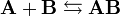# Dissociation constant

In biochemistry, chemistry and physics, the binding interaction of two molecules that bind with each other, for example a protein and a DNA duplex, is often quantified in terms of a dissociation constant, abbreviated as Kd, which is the inverse of the association constant, or Ka. The strength of the binding interaction is inversely proportional to Kd. Extremely tight-binding molecules such as antibodies and the their target exhibit Kd values in the picomolar range (10−12), while many drugs bind to their targets with Kd values in the nanomolar (10−9) to micromolar (10−6) range. Given the Kd of an interaction, and the initial concentrations of the interacting molecules, the amount of complex can be calculated.

##  Biomolecular Definition

Given two molecules, A and B, with initial molar concentrations [A]0 and [B]0, that form a reversible binding complex AB, having a certain dissociation constant Kd, that is,$\mathbf{A} + \mathbf{B} \leftrightarrows \mathbf{AB}$

The Kd, by definition, is$\mathbf{K_d} = \frac{\mathbf{[A]}\times\mathbf{[B]}}{\mathbf{[AB]}}$

Using the facts that [A] = [A]0 − [AB] and [B] = [B]0 − [AB] gives$\mathbf{K_d} = \frac{(\mathbf{[A]_0} - \mathbf{[AB]})\times(\mathbf{[B]_0} - \mathbf{[AB]})}{\mathbf{[AB]}}$

expanding the top terms yields$\mathbf{K_d} = \frac{\mathbf{[A]_0} \times \mathbf{[B]_0} - \mathbf{[A]_0} \times \mathbf{[AB]} - \mathbf{[B]_0} \times \mathbf{[AB]} + \mathbf{[AB]} \times \mathbf{[AB]}}{\mathbf{[AB]}}$

Multiplying both sides by [AB] and rearranging gives a quadratic equation:$\mathbf{[AB]^2} - \left(\mathbf{[A]_0} + \mathbf{[B]_0} + \mathbf{K_d}\right) \times \mathbf{[AB]} + \left(\mathbf{[A]_0} \times \mathbf{[B]_0}\right) = \mathbf{0}$

whose solution is:$\mathbf{[AB]} = \frac{(\mathbf{[A]_0} + \mathbf{[B]_0} + \mathbf{K_d}) \pm \sqrt{(\mathbf{[A]_0} + \mathbf{[B]_0} + \mathbf{K_d)^2} - \mathbf{4} \mathbf{[A]_0} \mathbf{[B]_0}}}{\mathbf{2}}$

Given the physical limitation that [AB] cannot be greater than either [A]0 or [B]0 eliminates the solution in which the square root term is added to the first term.

##  Implications

An inspection of the resulting solution shown above illustrates that in order to have an appreciable amount of bound material, the interacting molecules must be present at concentrations of 1/100 to 100 times the dissociation constant, as demonstrated in the table below, in which the concentrations of A and B are expressed in units of Kd.

[A]/Kd [B]/Kd %B bound
([AB]/[B])*100
0.001 0.001 0%
0.01 0.01 1%
0.1 0.1 8%
1.0 1.0 38%
10 10 73%
100 100 90%
1000 1000 97%Some content on this page may previously have appeared on Citizendium.